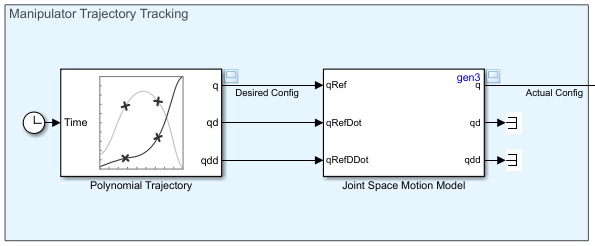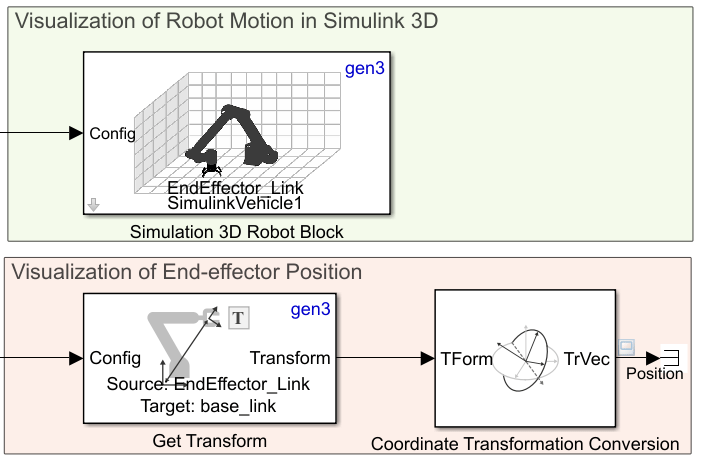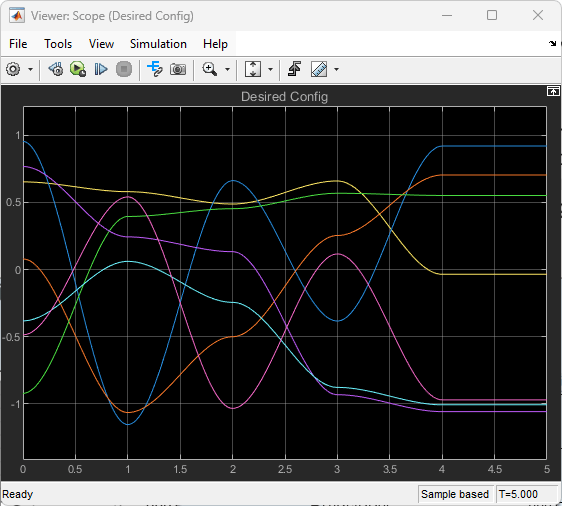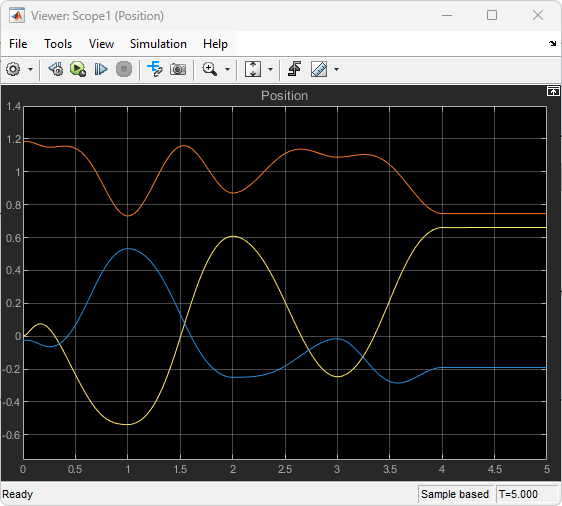# Visualize Manipulator Trajectory Tracking with Simulink 3D Animation

Simulate joint-space trajectories for a rigid body tree robot model and visualize the results with Simulink 3D Animation™.

### Model Overview

Load the model with the following command:

`open_system("SL3DJointSpaceManipulatorTrajectory")`This example uses a Kinova Gen3 manipulator, which is stored in the model workspace. However, load and visualize the robot with the following commands:

```gen3 = loadrobot("kinovaGen3","DataFormat","column"); show(gen3);```The model is split into two sections:

• Manipulator Trajectory Tracking

• Visualization in Simulink 3D Animation™

#### Manipulator Trajectory Tracking

The Polynomial Trajectory block generates continuous joint-space trajectories from random sets of waypoints in the range `[-0.375*pi 0.375*pi]`, stopping at each of the waypoints. The Joint-Space Motion Model block simulates the closed-loop tracking of these trajectories for a Kinova Gen3 manipulator with computed-torque control.#### Visualization in Simulink 3D Animation™

The VR RigidBodyTree block inserts the manipulator into the scene defined by the associated world file, `robot_manipulator_scene.wrl`. The VR Sink block provides a visualization for the world. In the block parameters, the VR Sink block has been modified to treat the setpoint, indicated by the red axes in the output, as an input. The Get Transform block is used to get the position of the end effector, which is then converted from a homogeoneous transform matrix to a translation vector, and then from MATLAB to VR coordinates.### Simulate the Model

`sim("SL3DJointSpaceManipulatorTrajectory.slx");`In the model, pacing is active, as indicated by the clock symbol below the run button:This ensures that the model is slowed down to near real-time speed, so that the visualization can be updated at a realistic pace.

#### Trajectory Visualization

By default, the model opens both the VR visualization and the scopes that display velocity and position information. However, if they are closed, the VR view can be reopened by clicking on the VR Sink block, and the scopes can be opened by double-clicking the associated viewer icons:The scopes show the tracking results of the Joint Space Motion Model block. As can be seen on the left in the figures below, the initial configuration of the robot differs from the reference trajectories, but the controlled motion ensures that the trajectory is reached and tracked for the duration of the simulation. The final scope displays the X, Y, and Z position of the end effector in the world frame.